Get inspired by the success stories of our students in IIT JAM MS, ISI  MStat, CMI MSc DS.  Learn More

#### IIT JAM MS 2020 Section A Problem 1 Solution

##### Problem

If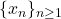is a sequence of real numbers such that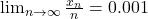, then

(A)is a bounded sequence
(B)is an unbounded sequence
(C)is a convergent sequence
(D)is a monotonically decreasing sequence

### Hints

##### Hint 1

Ifwas bounded, show that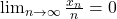, by sandwich theorem.

##### Hint 2

Ifwas convergent, show that, by algebra of limits.

##### Hint 3

Ifwas motonotically decreasing and bounded below, then it would have been convergent by Monotone Convergence Theorem.

Let's consider if it is not below below, i.e.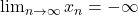• Take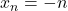• Take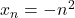• TakeFind the limit in each of this case.

Hence, it will be unbounded. See the full solution and proof idea below.

#### Food For Thoughts

• What if,?
• Find examples.

### Knowledge Partner